# 有意思的数字盲水印的简单的实现。

早期大约是10年前从一本数字图像处理上看到过数字水印的概念，觉得确实一种很有意思的东西，那个时候主要就是基于LSB的图像信息的隐藏，这种在空域里的方法有较大的缺陷，鲁棒性是比较差的。随便一个后期的都会造成水印的丢失，因此，虽然是一种盲水印，但是不具有很好的推广性。

前段时间一个朋友给了我一段使用Opencv的盲水印代码，是基于FFT变换的， 抽空看了下，对其中部分的实现过程进行了替换和分解，也实现了一个最简单的基于频域的盲水印效果。

我在寻找相关资料的时候在网络上看到有几个这方面的文章和工具，现在分享如下：

好像还有一个写的比较详细，而且有工具，在github上也有分享代码。

但是似乎这些工具大部分只支持文字水印，而不支持图像水印，文字我不熟悉，因此我还是用图像做水印模板，核心的代码如下所示：

int IM_AddBlindWaterMark(unsigned char *Src, unsigned char *WaterMark, unsigned char *Dest, int Width, int Height, int Stride, int WidthW, int HeightW, int StrideW)
{
int Channel = Stride / Width, ChannelW = StrideW / WidthW;
if ((Src == NULL) || (Dest == NULL))                        return IM_STATUS_NULLREFRENCE;
if ((Width <= 0) || (Height <= 0))                            return IM_STATUS_INVALIDPARAMETER;
if ((Channel != 1) && (Channel != 3) && (Channel != 4))        return IM_STATUS_INVALIDPARAMETER;
if ((ChannelW != 1) && (ChannelW != 3) && (ChannelW != 4))    return IM_STATUS_INVALIDPARAMETER;
if ((WidthW >= Width / 4) || (HeightW >= Height / 4))        return IM_STATUS_INVALIDPARAMETER;        //    水印图不能大于原图尺寸的一半

int Status = IM_STATUS_OK;

int OptimalW = IM_GetOptimalDftSize(Width),    OptimalH = IM_GetOptimalDftSize(Height);
int OffsetX = (OptimalW - Width) / 2,        OffsetY = (OptimalH - Height) / 2;
int HalfW = OptimalW / 2,                    HalfH = OptimalH / 2;

if (Channel == 1)
{
Complex    *Data = (Complex *)malloc(OptimalW * OptimalH * sizeof(Complex));
if ((Data == NULL))    return IM_STATUS_OUTOFMEMORY;

for (int Y = 0; Y < Height; Y++)                                //    我们把数据居中布置，边缘用重复像素的方式
{
unsigned char *LinePS = Src + Y * Stride;
Complex *LinePD = Data + (Y + OffsetY) * OptimalW;
for (int X = 0; X < OffsetX; X++)
{
LinePD[X].Real = LinePS;
LinePD[X].Imag = 0;
}
for (int X = OffsetX; X < OffsetX + Width; X++)
{
LinePD[X].Real = LinePS[X - OffsetX];
LinePD[X].Imag = 0;
}
for (int X = OffsetX + Width; X < OptimalW; X++)
{
LinePD[X].Real = LinePS[Width - 1];
LinePD[X].Imag = 0;
}
}
for (int Y = 0; Y < OffsetY; Y++)
{
memcpy(Data + Y * OptimalW, Data + OffsetY * OptimalW, OptimalW * sizeof(Complex));
}
for (int Y = OffsetY + Height; Y < OptimalH; Y++)
{
memcpy(Data + Y * OptimalW, Data + (OffsetY + Height - 1) * OptimalW, OptimalW * sizeof(Complex));
}

IM_FFT2D(Data, Data, OptimalW, OptimalH, false, 0, 0);
IM_FFTShift(Data, Data, OptimalW, OptimalH);                //    数据偏移到中心

for (int Y = 0; Y < HeightW; Y++)
{
Complex *LineLT = Data + (Y + OffsetY + Height / 16) * OptimalW + OffsetX + Width / 16;            //    确保在可见的范围内添加，左上角和右下角都镜像添加
Complex *LineRB = Data + (OffsetY + Height - 1 - Height / 16 - Y) * OptimalW + OffsetX + Width - 1 - Width / 16;    //    再稍微往内部移动一点，可以适当增强抵抗变形的能力，但是越往中心其对最终结果的影响越大。
unsigned char *LinePS = WaterMark + Y * StrideW;
if (ChannelW == 1)
{
for (int X = 0; X < WidthW; X++)
{
float Cof = ((LinePS[X] * 4) >> 8) + 1;
LineLT[X].Real *= Cof;    LineLT[X].Imag *= Cof;
LineRB[-X].Real *= Cof;    LineRB[-X].Imag *= Cof;
}
}
else if (ChannelW == 3)
{
for (int X = 0; X < WidthW; X++)
{
float Cof = ((((LinePS + LinePS + LinePS + LinePS) >> 2) * 4) >> 8) + 1;
LineLT[X].Real *= Cof;    LineLT[X].Imag *= Cof;
LineRB[-X].Real *= Cof;    LineRB[-X].Imag *= Cof;
LinePS += 3;
}
}
else if (ChannelW == 4)
{
for (int X = 0; X < WidthW; X++)
{
float Cof = ((IM_Div255(((LinePS + LinePS + LinePS + LinePS) >> 2) * LinePS) * 4) >> 8) + 1;
LineLT[X].Real *= Cof;    LineLT[X].Imag *= Cof;
LineRB[-X].Real *= Cof;    LineRB[-X].Imag *= Cof;
LinePS += 4;
}
}
}

IM_IFFTShift(Data, Data, OptimalW, OptimalH);
IM_FFT2D(Data, Data, OptimalW, OptimalH, true, 0, 0);

for (int Y = 0; Y < Height; Y++)
{
Complex *LinePS = Data + (Y + OffsetY) * OptimalW + OffsetX;
unsigned char *LinePD = Dest + Y * Stride;
for (int X = 0; X < Width; X++)
{
LinePD[X] = IM_ClampToByte(LinePS[X].Real);
}
}
if (Data != NULL)        free(Data);
return IM_STATUS_OK;
}
else
{

}
}

首先，把图像变换到频域，这里采用了opencv的有关FFT计算的过程，使用IM_GetOptimalDftSize计算最佳的DFT算法的大小，然后将图像数据居中分布，周边的空白像素采用镜像填充方式填充，虚部数据填0。

FFT变换完成后，对FFT数据进行移位，把高频数据放置到图像的中心，低频的数据放置到图像的边缘。为了将水印的图像嵌入到目标图像，我们在适当位置根据水印图像的强度或内容来修改这些频域值，为了不影响最终的目标图像的视觉效果，嵌入的数据放置到边缘的低频数据中（靠近边缘的部位）,我这里也没有放置在最边缘，而是边缘靠中的部位。

常用的水印图像可能是8位灰度、24位彩色或32位透明图，因此，我在程序里对不同位数的水印图都做了处理，如果是32位图，则把Alpha也考虑进去了，使用的嵌入方式就是最简单的更具水印图的颜色强度值将目标图像的频域系数放大。这里的放大程度我做了固定的设计，测试效果还比较好，如果过度放大，则最后处理的结果将会严重的失真，这就失去了算法本身的意义了。当然还有一种方式就是缩小系数，也可以去尝试下。

之后，我们需要将平移后的数据再次进行移位，然后就是进行IFFT计算了，并将计算结果返回到图像域。

本例只给出了针对灰度目标图像的代码，那么彩色图像其实是一样的过程，将他们分解成三个通道单独处理就OK了。

同时，为了保证水印对结果图不会造成太大的影响，我们程序对水印图大小做了限制，长和宽都不得大于目标图像的1/4。

另外，从嵌入的代码可以看到，我们希望水印图像尽量是黑色的背景（8位或24位）或纯背景部位是透明的（32位），这样对目标图像的影响也比较小。

我们来做一些测试，以下是一张原图(原图缩小显示了）及两个水印图进行测试：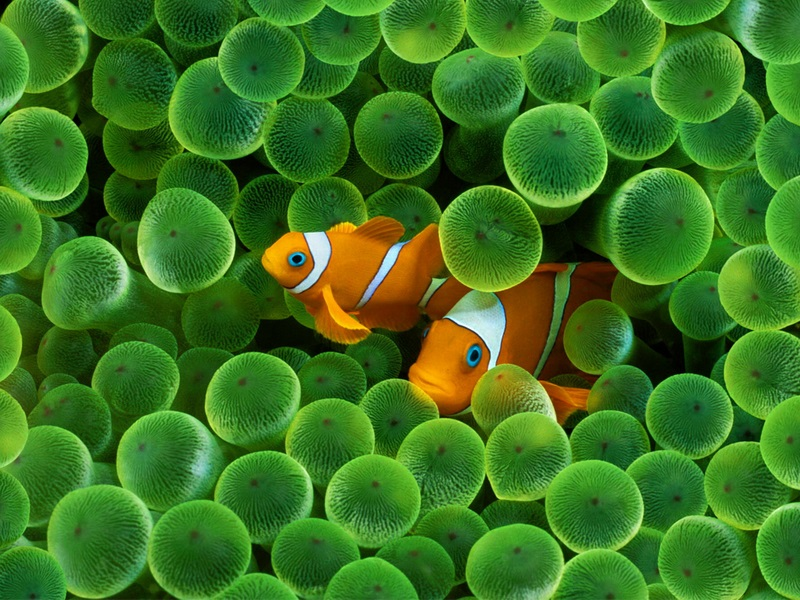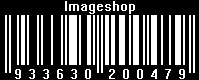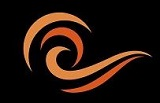分别查看其结果图和频谱图：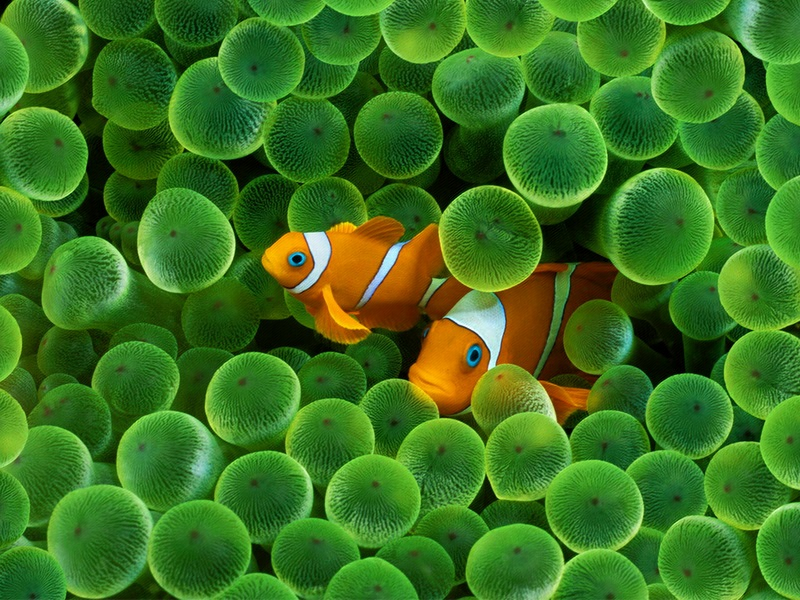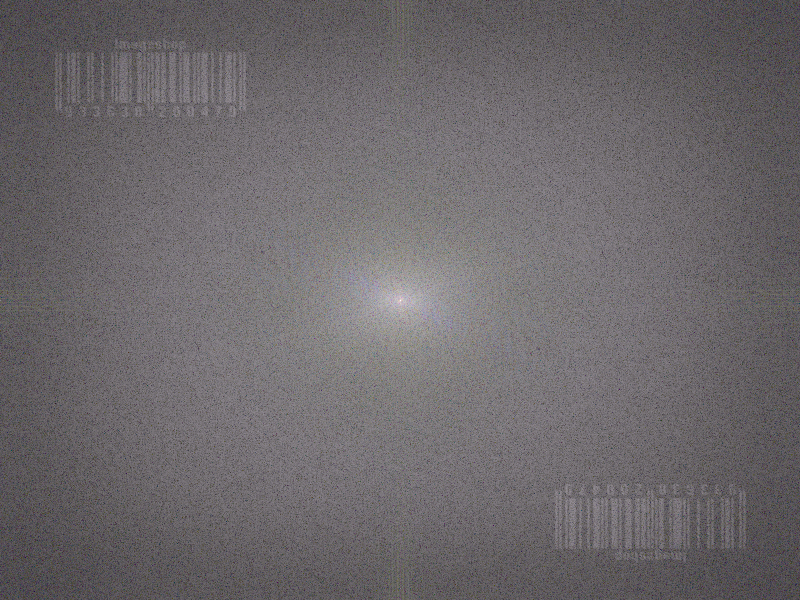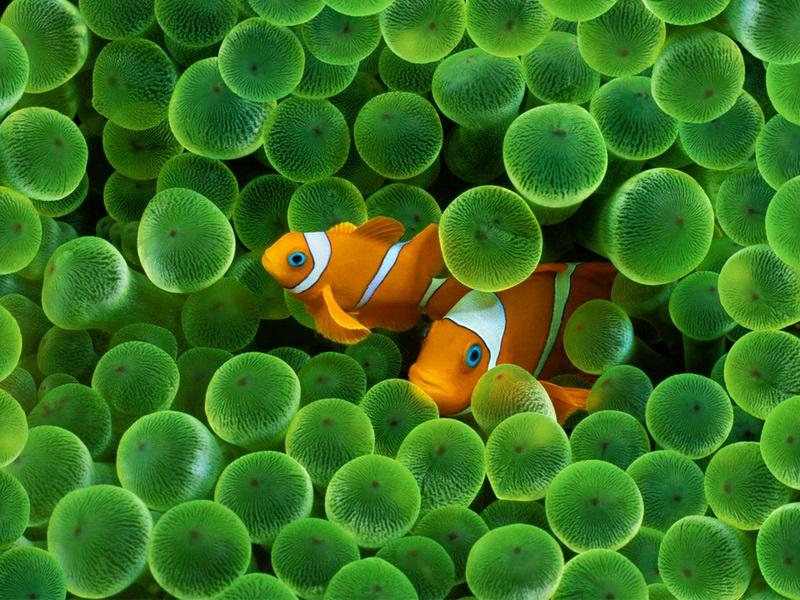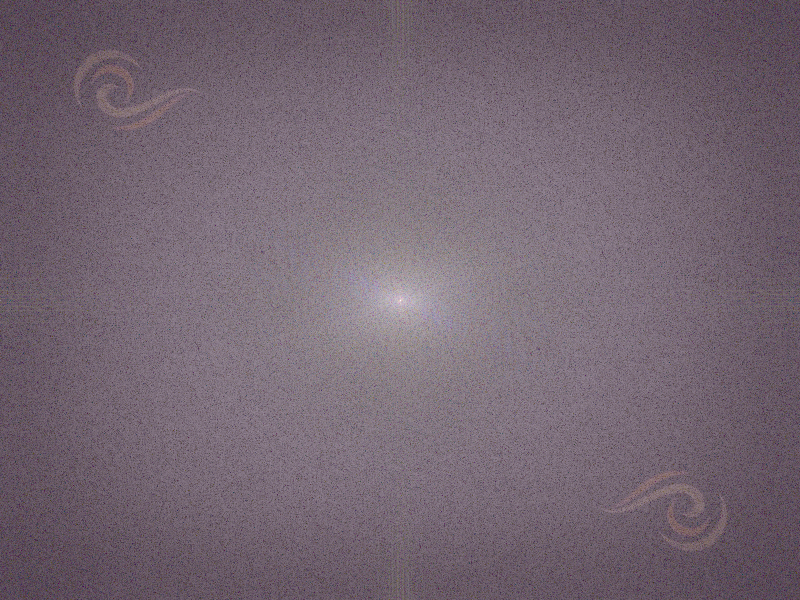可见，添加水印后基本未对原始图像造成视觉上的损失，在处理后的图像的频谱上可以明显看到添加后的水印的样式。

如果对添加水印后的图像进行一些处理，看看水印是否还能有效保存。

一、乱七八糟的增强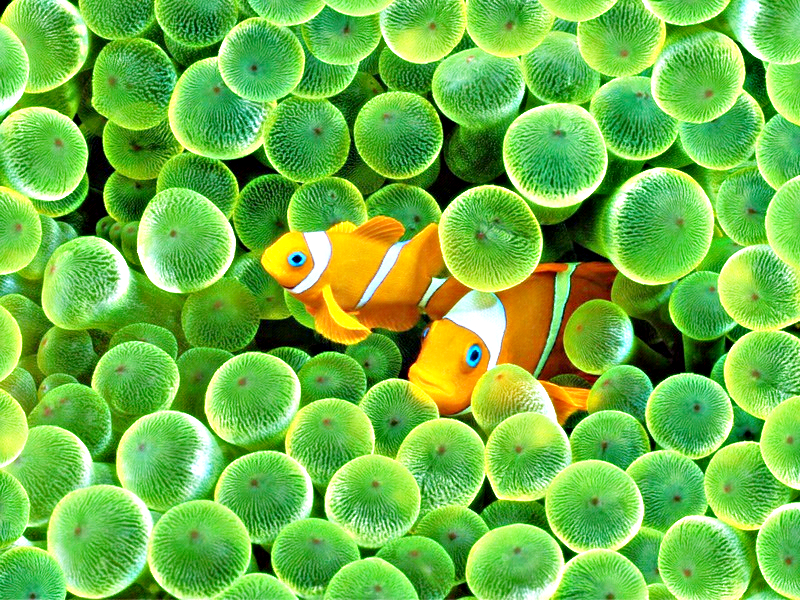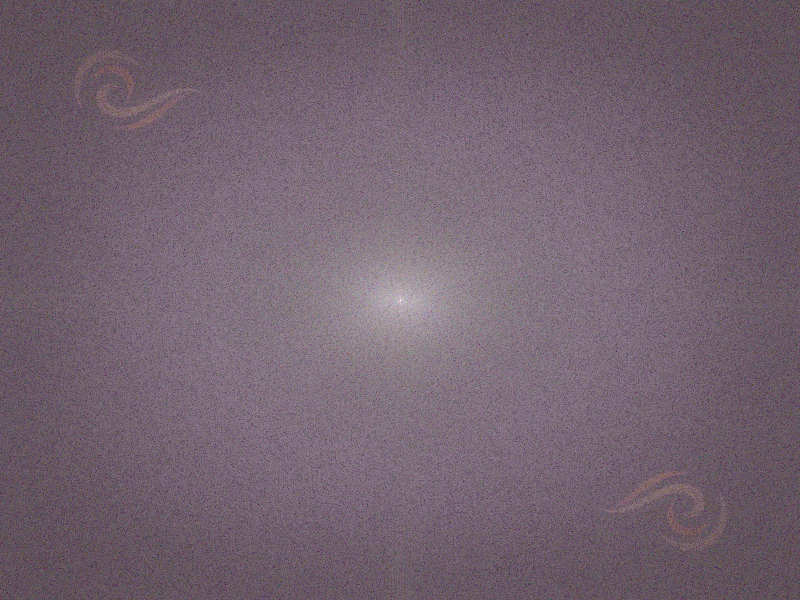2、有局部裁切的旋转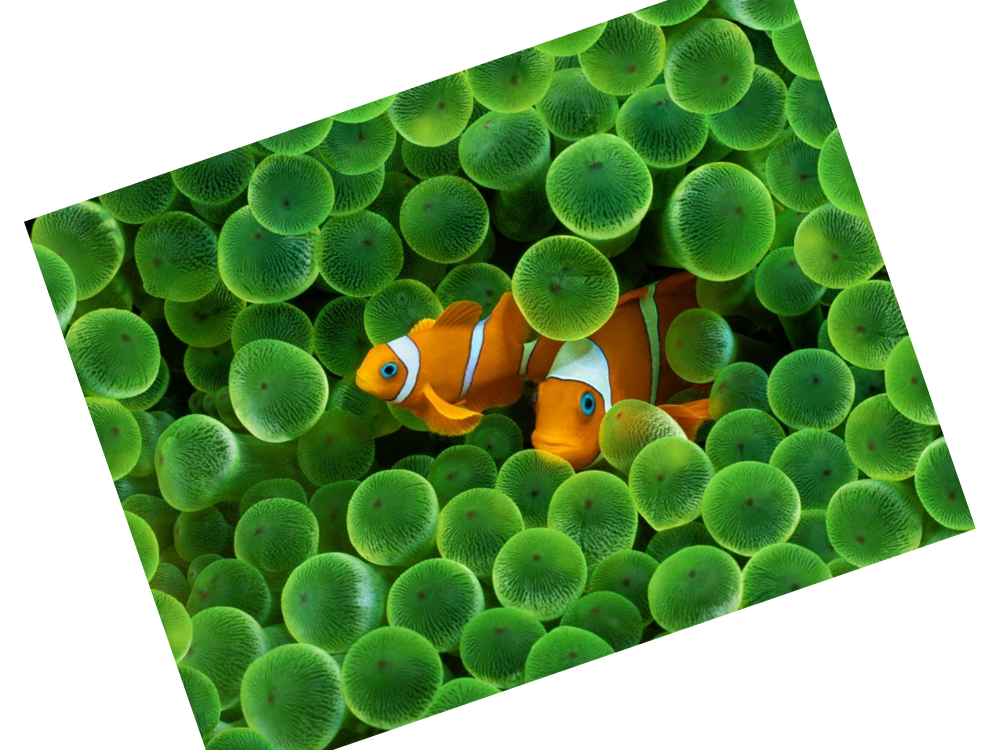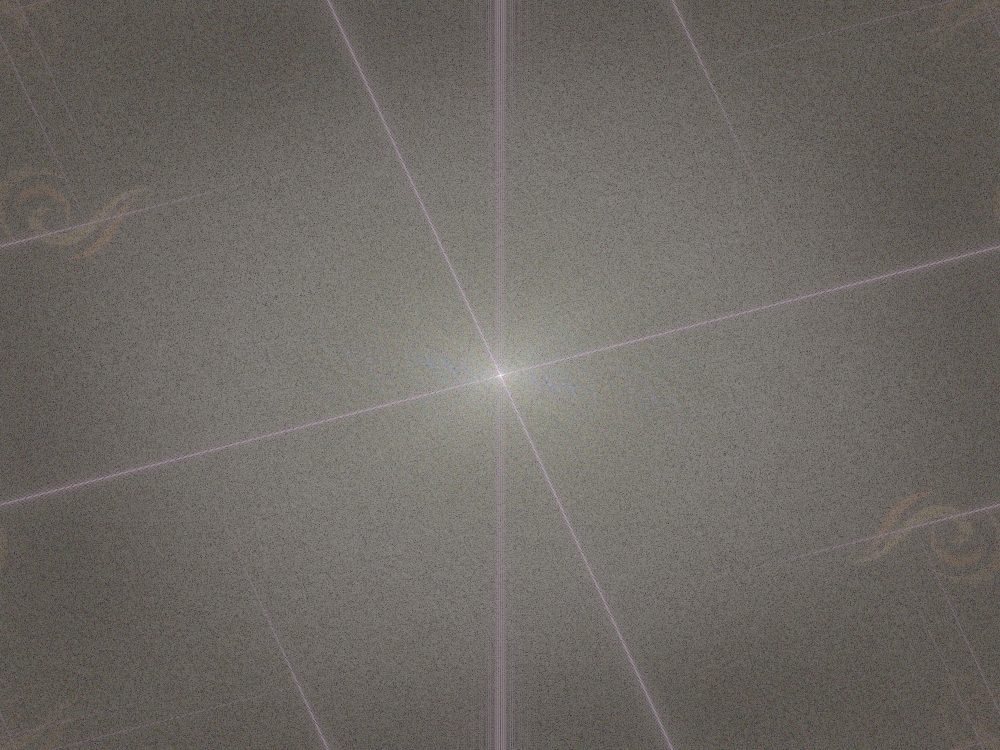3、含有模糊性质的算法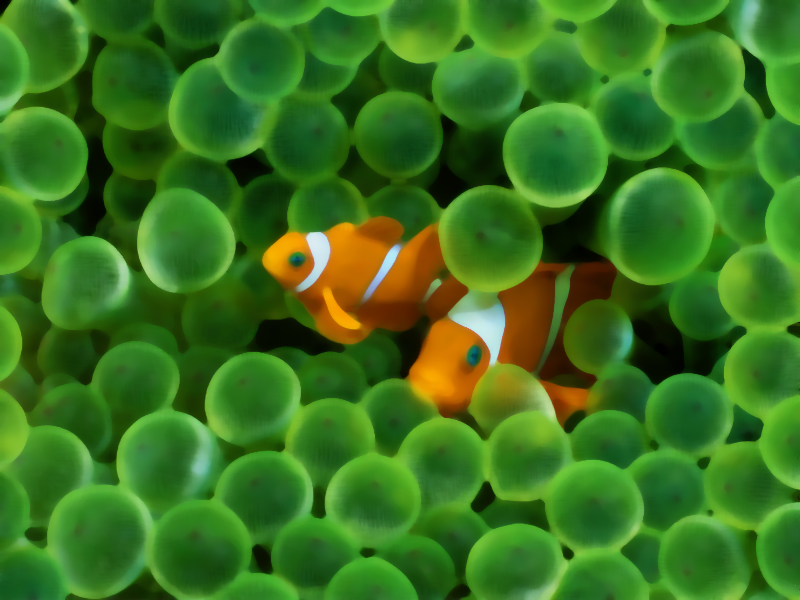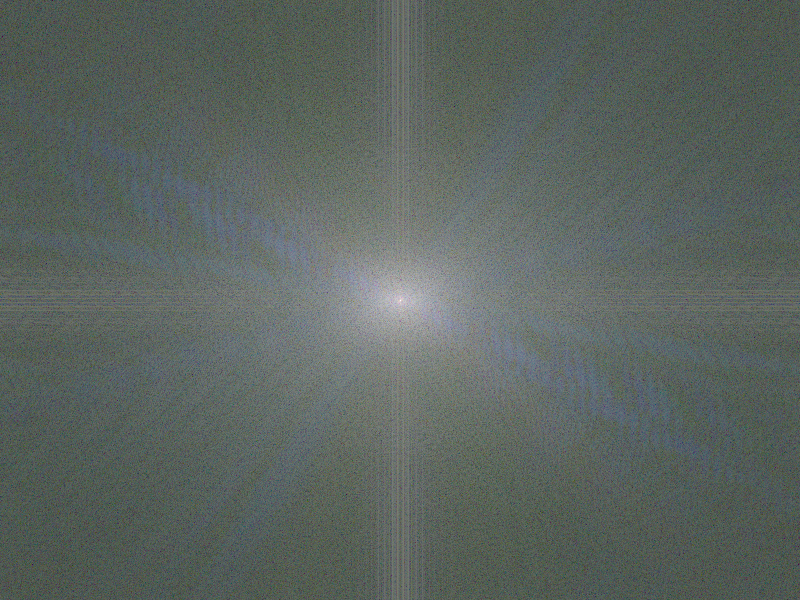可见，这个时候水印信息就基本丢失了，这主要是因为我们的水印信息是加在图像的低频的，而模糊会对低频进行处理，所以就看不到水印了，但是如果是锐化算法就不成问题的。

因此，这个盲水印的功能还是比较初级的，但是如果在自己的比较重要的图里隐藏个水印有的时候还是值得的，假如某个坏人是直接使用你的图而没有做任何更改呢。

另外，还有一种基于FFT比较常见的水印技术，需要嵌入水印的图片以及未嵌入水印的原始图这样才可以获得水印，理论上讲这种应该不叫做盲水印了，但是他有个好处就是可以对水印进行加密，这样别人就比较难以知道你对图像是否嵌入了水印了。需要做的额外工具就是一定需要保留原始的未加水印的图像了。

我将这个 小工具也集成到了我的SSE做的DEMO里了，有需要的朋友可以试下：SSE_Optimization_Demo.rar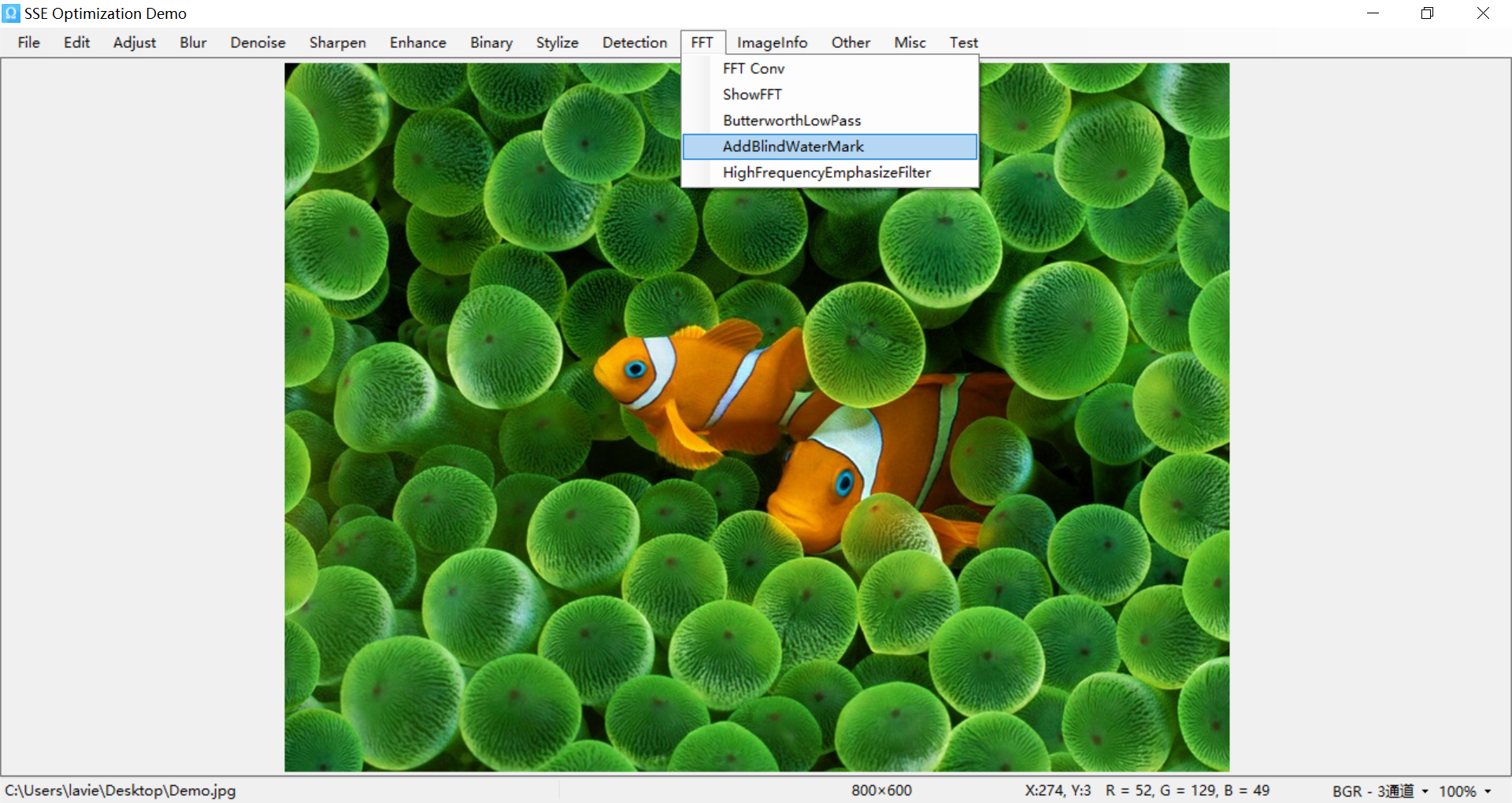posted @ 2018-12-10 18:37  Imageshop  阅读(11231)  评论(3编辑  收藏  举报